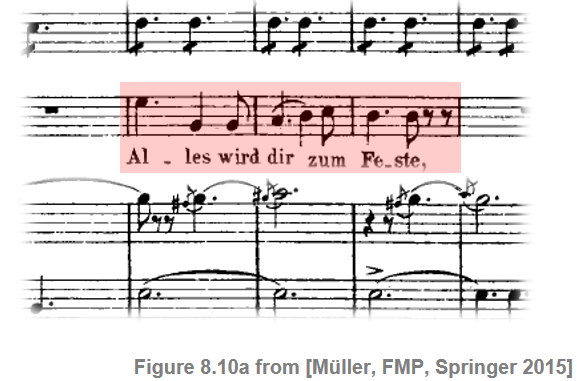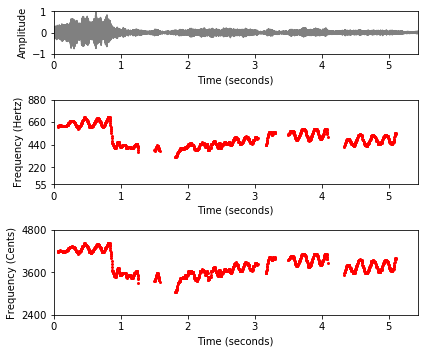# Fundamental Frequency Tracking

Following Section 8.2.3 of [Müller, FMP, Springer 2015], we discuss in this notebook various variants for tracking the fundamental frequency.

## Frequency Trajectory¶

In general terms, a melody may be defined as a linear succession of musical tones that form a coherent entity and express a particular musical idea. As with many other concepts in music processing, the notion of melody remains rather vague (see the FMP notebook on melody extraction and separation for a more detailed discussion). In this notebook, we consider the scenario where the music is given in the form of an audio recording (and not as a symbolic music representation). Furthermore, rather than estimating a sequence of notes, our objective is to determine a sequence of frequency values that correspond to the notes' pitches. Such a frequency path over time, which may also capture continuous frequency glides and modulations, is referred to as a frequency trajectory. In particular, we are interested in the fundamental frequency values (also called F0-values) of the melody's notes. The resulting trajectory is also called an F0-trajectory. Mathematically, we model an F0-trajectory to be a function

\begin{equation} \eta:\mathbb{R}\to\mathbb{R}\cup\{\ast\}, \end{equation}

which assigns to each time point $t\in\mathbb{R}$ (given in seconds) either a frequency value $\eta(t)\in\mathbb{R}$ (given in Hertz) or the symbol $\eta(n)=\ast$. The interpretation of $\eta(t)=\ast$ is that there is no F0-value corresponding to the melodic component at this time instance.

As an example, we consider a short excerpt of an aria from the opera "Der Freischütz" by Carl Maria von Weber, which we already used in the FMP notebook on salience representations. In the score representation, the main melody is notated in a separate staff line underlaid with lyrics. In a performance by a soprano singer, the melody corresponds to a trajectory of F0-values. As opposed to the notated symbolic representation, some of the notes are smoothly connected. Furthermore, one can observe rather pronounced frequency modulations due to vibrato.In the following figure, we visualize the F0-trajectory of the singer (read from an annotation file), one with a linear frequency axis (given in Hertz) and once with a logarithmic frequency axis (given in cents relative to the reference frequency $\omega_\mathrm{ref}=55~\mathrm{Hz}$.

In :
import numpy as np
import os, sys, librosa
import pandas as pd
from scipy import signal
from scipy import linalg
import matplotlib
from matplotlib import pyplot as plt
from matplotlib.colors import ListedColormap
import IPython.display as ipd
from numba import jit

sys.path.append('..')
import libfmp.b
import libfmp.c3
import libfmp.c8
%matplotlib inline

def hz_to_cents(F, F_ref=55.0):
"""Converts frequency in Hz to cents

Notebook: C8/C8S2_FundFreqTracking.ipynb

Args:
F (float or np.ndarray): Frequency value in Hz
F_ref (float): Reference frequency in Hz (Default value = 55.0)

Returns:
F_cent (float or np.ndarray): Frequency in cents
"""
F_cent = 1200 * np.log2(F / F_ref)
return F_cent

def cents_to_hz(F_cent, F_ref=55.0):
"""Converts frequency in cents to Hz

Notebook: C8/C8S2_FundFreqTracking.ipynb

Args:
F_cent (float or np.ndarray): Frequency in cents
F_ref (float): Reference frequency in Hz (Default value = 55.0)

Returns:
F (float or np.ndarray): Frequency in Hz
"""
F = F_ref * 2 ** (F_cent / 1200)
return F

fn_wav = os.path.join('..', 'data', 'C8', 'FMP_C8_F10_Weber_Freischuetz-06_FreiDi-35-40.wav')
Fs = 22050
x_duration = len(x)/Fs
ipd.Audio(x, rate=Fs)

# Read in the F0 trajectory
fn_traj = os.path.join('..', 'data', 'C8', 'FMP_C8_F10_Weber_Freischuetz-06_FreiDi-35-40_F0-user-Book.csv')
traj = traj_df.values

fig, ax = plt.subplots(3, 1, gridspec_kw={'height_ratios': [1, 2, 2]}, figsize=(6,5))
libfmp.b.plot_signal(x, Fs, ax=ax, xlabel='');
ax.set_ylim((-1,1));
ax.set_ylabel('Amplitude')
ax.set_xlabel('Time (seconds)')

traj_plot = traj[traj[:, 1]>0]
ax.plot(traj_plot[:, 0], traj_plot[:, 1], color='r', markersize=4, marker='.', linestyle='');
ax.set_ylim((55,880));
ax.set_yticks([55, 220, 440, 660, 880])
ax.set_xlim((0, x_duration));
ax.set_ylabel('Frequency (Hertz)')
ax.set_xlabel('Time (seconds)')
plt.tight_layout()

ax.plot(traj_plot[:, 0], hz_to_cents(traj_plot[:, 1]), color='r', markersize=4, marker='.', linestyle='');
ax.set_ylim((2400, 4800));
ax.set_yticks([2400, 3600, 4800])
ax.set_xlim((0, x_duration));
plt.xlabel('Time (seconds)')
ax.set_ylabel('Frequency (Cents)')
plt.tight_layout()## Sonification of Frequency Trajectories¶

In the following code cell, we provide a function for sonifying a given frequency trajectory using sinusoids.

In :
def sonify_trajectory_with_sinusoid(traj, audio_len, Fs=22050, amplitude=0.3, smooth_len=11):
"""Sonification of trajectory with sinusoidal

Notebook: C8/C8S2_FundFreqTracking.ipynb

Args:
traj (np.ndarray): F0 trajectory (time in seconds, frequency in Hz)
audio_len (int): Desired audio length in samples
Fs (scalar): Sampling rate (Default value = 22050)
amplitude (float): Amplitude (Default value = 0.3)
smooth_len (int): Length of amplitude smoothing filter (Default value = 11)

Returns:
x_soni (np.ndarray): Sonification
"""
# unit confidence if not specified
if traj.shape < 3:
confidence = np.zeros(traj.shape)
confidence[traj[:, 1] > 0] = amplitude
else:
confidence = traj[:, 2]

# initialize
x_soni = np.zeros(audio_len)
amplitude_mod = np.zeros(audio_len)

# Computation of hop size
# sine_len = int(2 ** np.round(np.log(traj[1, 0]*Fs) / np.log(2)))
sine_len = int(traj[1, 0] * Fs)

t = np.arange(0, sine_len) / Fs
phase = 0

# loop over all F0 values, insure continuous phase
for idx in np.arange(0, traj.shape):
cur_f = traj[idx, 1]
cur_amp = confidence[idx]

if cur_f == 0:
phase = 0
continue

cur_soni = np.sin(2*np.pi*(cur_f*t+phase))
diff = np.maximum(0, (idx+1)*sine_len - len(x_soni))
if diff > 0:
x_soni[idx * sine_len:(idx + 1) * sine_len - diff] = cur_soni[:-diff]
amplitude_mod[idx * sine_len:(idx + 1) * sine_len - diff] = cur_amp
else:
x_soni[idx*sine_len:(idx+1)*sine_len-diff] = cur_soni
amplitude_mod[idx*sine_len:(idx+1)*sine_len-diff] = cur_amp

phase += cur_f * sine_len / Fs
phase -= 2 * np.round(phase/2)

# filter amplitudes to avoid transients
amplitude_mod = np.convolve(amplitude_mod, np.hanning(smooth_len)/np.sum(np.hanning(smooth_len)), 'same')
x_soni = x_soni * amplitude_mod
return x_soni

x_traj_mono = sonify_trajectory_with_sinusoid(traj, len(x), Fs, smooth_len=11, amplitude=0.6)
# left: x, right: sonification
x_traj_stereo = np.vstack((x.reshape(1,-1), x_traj_mono.reshape(1,-1)))

print('F0 sonification (mono)')
ipd.display(ipd.Audio(x_traj_mono, rate=Fs))
print('F0 sonification superimposed with original recording (mono)')
ipd.display(ipd.Audio( (x_traj_mono+x)/2, rate=Fs))
print('F0 sonification (right channel), original recording (left channel)')
ipd.display(ipd.Audio(x_traj_stereo, rate=Fs))

F0 sonification (mono)

F0 sonification superimposed with original recording (mono)

F0 sonification (right channel), original recording (left channel)